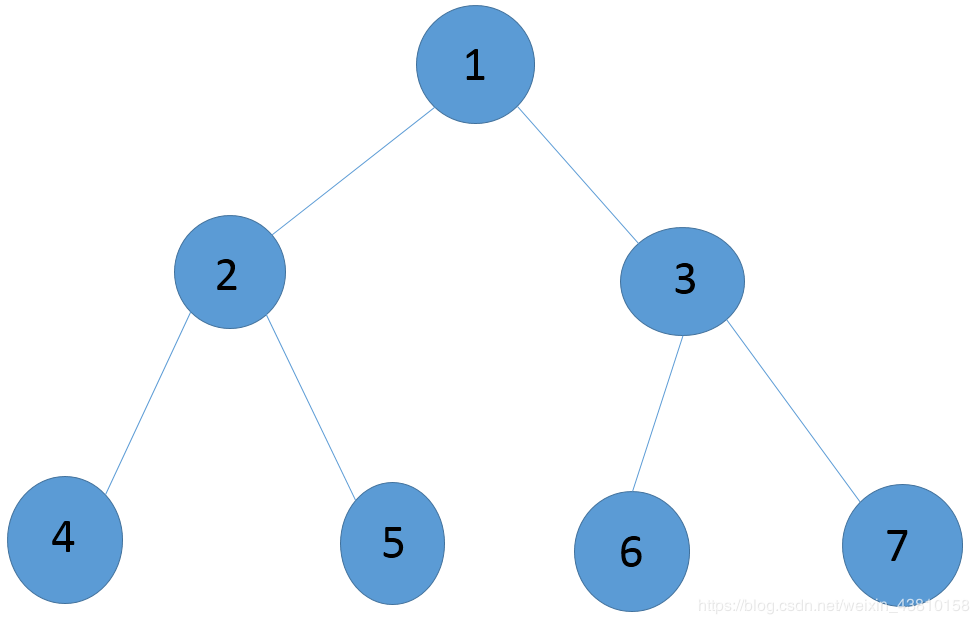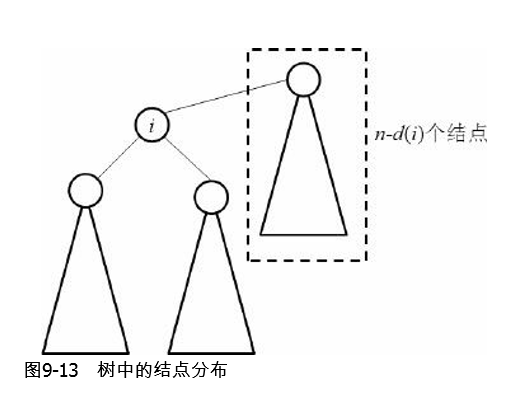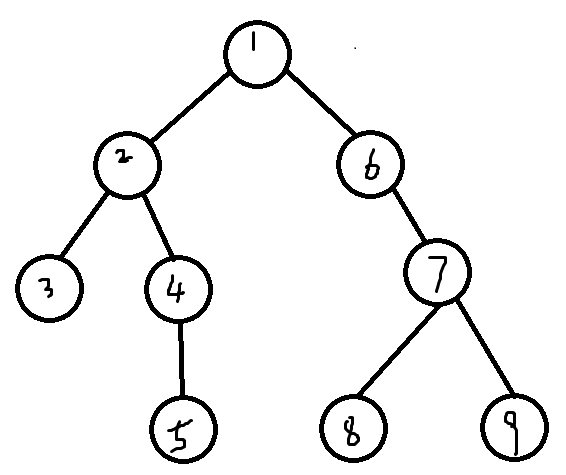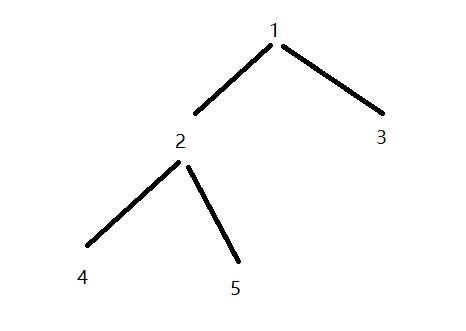• 树的重心也叫树的质心。对于一棵树n个节点的无根树，找到一个点，使得把树变成以该点为根的有根树时，最大子树的结点数最小。 树的重心定义为树的某个节点，当去掉该节点后，树的各个连通分量中，节点数最多的连通...
目录定义：性质：算法分析：POJ 1655  Balancing Act（求重心）POJ 3107 GodfatherP1364 医院设置（树形DP）
定义：
树的重心也叫树的质心。对于一棵树n个节点的无根树，找到一个点，使得把树变成以该点为根的有根树时，最大子树的结点数最小。
树的重心定义为树的某个节点，当去掉该节点后，树的各个连通分量中，节点数最多的连通分量其节点数达到最小值。树可能存在多个重心。如下图，当去掉点1后，树将分成两个连通块：（2,4,5），（3,6,7），则最大的连通块包含节点个数为3。若去掉点2，则树将分成3个部分，（4），（5），（1,3,6,7）最大的连通块包含4个节点；第一种方法可以得到更小的最大联通分量。可以发现，其他方案不可能得到比3更小的值了。所以，点1是树的重心。来源博客
性质：

树上所有的点到树的重心的距离之和是最短的，如果有多个重心，那么总距离相等。
把两棵树通过一条边相连，新的树的重心在原来两棵树重心的连线上。
一棵树添加或者删除一个节点，树的重心最多只移动一条边的位置。
一棵树最多有两个重心，且相邻。

算法分析：
和树的最大独立问题类似，先任选一个结点作为根节点，把无根树变成有根树，然后设 $d[i]$ 表示以i为根的子树的结点的个数。不难发现$d[i]=∑d[j]+1$，$j∈s[i]$。$s[i]$为i结点的所有儿子结点的编号的集合。程序也十分简单：只需要DFS一次，在无根树转有根数的同时计算即可，连记忆化都不需要——因为本来就没有重复计算。
那么，删除结点i后，最大的连通块有多少个呢？结点i的子树中最大有$max({d[j]})$个结点，i的“上方子树”中有$n-d（i）$个结点，这样，在动态规划的过程中就可 以顺便找出树的重心了。以上内容来自《算法竞赛入门经典》
POJ 1655  Balancing Act（求重心）
Balancing Act
题意:给定一棵树,求树的重心的编号以及重心删除后得到的最大子树的节点个数size,如果size相同就选取编号最小的.
Sample Input
1
7
2 6
1 2
1 4
4 5
3 7
3 1

Sample Output
1 2

#include<string.h>
#include<iostream>
#include<cstdio>
#include<algorithm>
#include<vector>
using namespace std;
typedef long long ll;
typedef pair<double,ll>pdl;
#define debug(x) cerr<<"# "<<x<<endl
const ll N=20005;
const ll base=137;
const ll mod=2147483647;
const int INF = 1<<30;
//const double INF=double(INT_MAX*1.0);
ll head[N];//链式前向星
ll son[N];//son[i]表示以i为根的子树节点个数
ll cnt,n;
ll ans,size;
bool vis[N];//代替了其他做法里的fa  (father)
struct Edge//链式前向星
{
ll to;
ll nex;
};
Edge edge[2*N];
inline void init()//初始化
{
cnt=0;
size=INF;
memset(vis,0,sizeof vis);
memset(head,-1,sizeof head);
}
inline void add(ll u,ll v)//链式前向星
{
edge[cnt].to=v;
edge[cnt].nex=head[u];
head[u]=cnt++;
}
inline void dfs(ll cur)
{
vis[cur]=1;
son[cur]=1;//节点本身
ll tmp=0;//tmp为节点cur的最大子树节点个数
for(ll i=head[cur];~i;i=edge[i].nex)
{
ll v=edge[i].to;
if(!vis[v])//if(v!=fa)
{
dfs(v);
son[cur]+=son[v];
tmp=max(tmp,son[v]);//同上
}
}
tmp=max(tmp,n-son[cur]);//更新,最大子树的节点个数size
if(tmp<size||(tmp==size&&cur<ans))//更新，要最小的那一个才是重心
{
ans=cur;
size=tmp;
}
}
int main()
{
ll T;
scanf("%lld",&T);
while(T--)
{
init();
scanf("%lld",&n);
for(int i=1;i<=n-1;++i)
{
ll u,v;
scanf("%lld %lld",&u,&v);
add(u,v);//无向图必须双向
add(v,u);
}
dfs(1);
printf("%lld %lld\n",ans,size);
}
return 0;
}


POJ 3107 Godfather
Godfather
题意:给定一棵树,求树的所有重心,按照编号从小到大的顺序输出.
分析:本题与上题基本上一样,只是求的量不同,既然我们在找树的重心的时候用的树型dp,而且是求的子树中节点数的最大值,然后求所有最大值的最小值,那么就有可能存在多个重心,我们每更新到一个最小值的时候就记录其它的最小值也为这个最小值的重心,这样下去就会找到所有的重心.
#include <iostream>
#include <string.h>
#include <algorithm>
#include <stdio.h>

using namespace std;
const int N = 50005;
const int INF = 1<<30;

int head[N];
int son[N];
bool vis[N];
int cnt,n;
int num,size;
int ans[N];

struct Edge
{
int to;
int next;
};

Edge edge[2*N];

void Init()
{
cnt = 0;
num = 0;
size = INF;
memset(vis,0,sizeof(vis));
memset(head,-1,sizeof(head));
}

void add(int u,int v)
{
edge[cnt].to = v;
edge[cnt].next = head[u];
head[u] = cnt++;
}

void dfs(int cur)
{
vis[cur] = 1;
son[cur] = 0;
int tmp = 0;
for(int i=head[cur];~i;i=edge[i].next)
{
int u = edge[i].to;
if(!vis[u])
{
dfs(u);
son[cur] += son[u] + 1;
tmp = max(tmp,son[u] + 1);
}
}
tmp = max(tmp,n-son[cur]-1);
if(tmp < size)
{
num = 1;
ans = cur;
size = tmp;
}
else if(tmp == size)
{
ans[num++] = cur;
}
}

int main()
{
while(~scanf("%d",&n))
{
Init();
for(int i=1;i<=n-1;i++)
{
int u,v;
scanf("%d%d",&u,&v);
add(u,v);
add(v,u);
}
dfs(1);
sort(ans,ans+num);
for(int i=0;i<num;i++)
printf("%d ",ans[i]);
puts("");
}
return 0;
}

P1364 医院设置（树形DP）
洛谷P1364 医院设置
定义几个数组：$f[u]$表示以u为根的总距离，$size[u]$表示以u为根的子树的大小（结点数，此题每个点要乘以权值，下文结点数均指此）。
显然，$ans=min(f[i],1<=i<=n)$
首先我们任意以一个点为根dfs一遍，求出以该点为根的总距离。方便起见，我们就以1为根。
接下来就是转移，对于每个u能达到的点v，有：
$f[v]=f[u]+size−size[v]−size[v]$
怎么来的呢？试想，当根从u变为v的时候，v的子树的所有节点原本的距离要到u，现在只要到v了，每个结点的距离都减少1，那么总距离就减少$size[v]$，同时，以v为根的子树以外的所有节点，原本只要到u就行了，现在要到v，每个节点的路程都增加了1，总路程就增加了$size−size[v]$，其中$size$就是我们预处理出来的整棵树的大小，减去$size[v]$就是除以v为根的子树以外的结点数。
最后取最小值，得解。时间复杂度$O(n)$
上述思路来源
#include<string.h>
#include<iostream>
#include<cstdio>
#include<algorithm>
#include<vector>
using namespace std;
typedef long long ll;
typedef pair<double,ll>pdl;
#define debug(x) cerr<<"# "<<x<<endl
const ll N=20005;
const ll base=137;
const ll mod=2147483647;
const int INF = 1<<30;
struct Edge
{
ll to;
ll nex;
}tree[N];
ll head[N],cnt,w[N],n,size[N];
ll ans=INF,f[N];
inline void add(ll u,ll v)//链式前向星建树
{
tree[++cnt].to=v;
tree[cnt].nex=head[u];
head[u]=cnt;
}
inline void init()//初始化
{
memset(head,-1,sizeof head);
cnt=0;
}
inline void dfs(ll u,ll fa,ll dep)//以u为根，father为fa，深度为dep
{
size[u]=w[u];//size[u]表示以u为根的子树的大小(结点数，此题每个点要乘以权值，正常情况下应该是1)
for(int i=head[u];~i;i=tree[i].nex)//遍历所有相连的子树
{
if(tree[i].to!=fa)
{
dfs(tree[i].to,u,dep+1);//往下走
size[u]+=size[tree[i].to];
}
}
f+=w[u]*dep;//f[u]表示以u为根的总距离，先预处理求得以1为根，f的值
}
inline void dp(ll u,ll fa)
{
for(int i=head[u];~i;i=tree[i].nex)
{
if(tree[i].to!=fa)//防止自环
{
//通过转移方程直接把所有的点当作根的deep算出来然后比较
f[tree[i].to]=f[u]+size-size[tree[i].to]*2;
dp(tree[i].to,u);
}
}
ans=min(ans,f[u]);
}
int main()
{
init();
scanf("%lld",&n);
for(int i=1;i<=n;++i)
{
ll a,b;
scanf("%lld",&w[i]);
scanf("%lld %lld",&a,&b);
if(a)add(i,a),add(a,i);
if(b)add(i,b),add(b,i);
}
dfs(1,0,0);
dp(1,0);
printf("%lld\n",ans);
return 0;
}




展开全文• 1.树的重心 树的重心是指树上一点，去掉后最大子树可以取得最小值的点。 这样定义可能比较抽象，我们来看一个例子—— 【无根树】 去掉1后，子树最大为4；去掉2后，子树最大为5；去掉3后，没影响；去掉4后，...
初见安~这里讲述的树上操作真的都是非常非常基础的哈：）都是要掌握的。

1.树的重心

树的重心是指树上一点，去掉后最大子树可以取得最小值的点。

这样定义可能比较抽象，我们来看一个例子——【无根树】

去掉1后，子树最大为4；去掉2后，子树最大为5；去掉3后，没影响；去掉4后，子树最大为7；去掉5后，没影响；去掉6后，子树最大为5；去掉7后，子树最大为6；去掉8、9后，均无影响。所以这棵树的重心为点1。

由此也可以得出重心的另一个定义——去掉该点后最大子树大小不超过n/2。这一定义在应用方面比较多。

看看代码就可以大概理解了——

#include<bits/stdc++.h>
#define maxn 20005
using namespace std;
int n;
struct edge
{
int to, nxt;
edge(){}
edge(int tt, int nn)
{
to = tt, nxt = nn;
}
}e[maxn << 1];

int head[maxn], k = 0;
void add(int u, int v)
{
e[k] = edge(v, head[u]);
head[u] = k++;
}

bool vis[maxn];
int size[maxn], ans = 0x3f3f3f3f;
int maxpart[maxn];
void dfs(int x)
{
vis[x] = 1, size[x] = 1;
maxpart[x] = 0;
for(int i = head[x]; ~i; i = e[i].nxt)
{
int y = e[i].to;
if(vis[y]) continue;
dfs(y);
size[x] += size[y];
maxpart[x] = max(maxpart[x], size[y]);//这是x的子树
}

maxpart[x] = max(maxpart[x], n - size[x]);//这是x的另一颗子树（无根树，换根法
if(maxpart[x] < ans)
{
ans = maxpart[x];
}
}
int main()
{
memset(head, -1, sizeof head);
scanf("%d", &n);
int u, v;
for(int i = 1; i < n; i++)
{
scanf("%d%d", &u, &v);
add(u, v);
add(v, u);
}

dfs(1);
for(int i = 1; i <= n; i++)
{
if(maxpart[i] == ans) printf("%d\n", i);
}
return 0;
}

2.树的直径

树的直径的定义相对而言要简单一些——就是树上最远两点之间的距离。

比方说还是方才那个图——最远的两点5、8或者5、9之间的距离为6。6就是这棵树的直径。

那么我们怎么求树的直径呢——因为我们不知道直径的两端分别是哪两个点。所以我们可以先随便找个点，找到离他最远的点，再在那个最远的点上找一次最远的点，这两个点之间的距离就是直径。比如上图，我们从1开始，最远的是5（或者8、9），从5开始找，最远的点就是8or9了。

证明如下——我们可以发现，最远的两点一定不在根节点的同一子树上，在此基础上各自深度为这棵树的最深。也就是说——既然是最深的点，那么对于其他普通点来说，最远的点也一定是到达了叶子节点的，所以一定会找到一个非同一子树的深度最深的叶子节点。而这个点就一定是我们直径两端的其中一点。再从这个点出发找到最远，就一定是树的直径了。

所以我们两次bfs找最远就可以了。

代码实现如下——

#include<bits/stdc++.h>
#define maxn 100005
using namespace std;
int n, m;
int dep[maxn], dis[maxn], l = 1, r = 0;
int res, ans;//res为找到的最远点，ans为直径
bool vis[maxn];
struct edge
{
int to, w, nxt;
edge(){};
edge(int tt, int ww, int nn)
{
to = tt, w = ww, nxt = nn;
}
}e[maxn << 1];

int k = 0, head[maxn];
void add(int u, int v, int w)
{
e[k] = edge(v, w, head[u]);
head[u] = k++;
}

void bfs(int x)
{
memset(dis, 0, sizeof dis);//调用两次，必须清空
memset(vis, 0, sizeof vis);
queue<int> q;
q.push(x);
vis[x] = 1;
while(q.size())
{
int p = q.front();
q.pop();
if(dis[p] > ans)//找到了距离更远的
ans = dis[p], res = p;//可以直接更新
for(int i = head[p]; ~i; i = e[i].nxt)
{
int y = e[i].to, w = e[i].w;
if(!vis[y])
{
vis[y] = 1;
dis[y] = dis[p] + w;//计算dis，在下一次取出来时再和ans作比较
q.push(y);
}
}
}

}

int main()
{
memset(head, -1, sizeof head);
scanf("%d%d", &n, &m);
int u, v, w;
char op;
for(int i = 1; i <= m; i++)
{
scanf("%d%d%d %c", &u, &v, &w, &op);
add(u, v, w);
add(v, u, w);
}

bfs(1);//随便一个点
ans = 0;
bfs(res);//第二次
printf("%d\n", ans);
return 0;
}

3.例题

扫雪系列1

Description

大雪履盖了整个城市，市政府要求冬季服务部门尽快将一些街道（列在一份清单中）的积雪清除掉以恢复交通，整个城市由许多交叉路口和街道构成，当然任意两个交叉路口都是直接或间接连通的，清单给出了最少的街道，使得这些街道的积雪清除后任意两个交叉路口之间有且仅有一条通路，冬季服务部门只有一辆铲雪车及一名司机，这辆铲雪车的出发点位于某个交叉路口。
无论街道上有没有积雪，铲雪车每前进一米都要消耗一升燃料，冬季服务部门要求司机在铲除清单上的所有街道的积雪的前提下，要求消耗燃料最少，积雪铲完后车可以停在任意的交叉路口。

Input

输入文件的第一行包含两个整数N和S，1≤N≤100000，1≤S≤N。N为交叉路口的总数；S为铲雪车出发的路口序号。路口的标号为1••N。
接下来的N-1行为清单上的街道，每一行包含三个用空格隔开的整数A、B、C，表示一条从交叉路口A到交叉路口B的街道，C为该街道的长度，单位为米，1≤C≤1000。

Output

输出文件仅一行包含一个整数表示清除所有积雪所需的最少的燃料数量。

Sample Input

5  1
1  2  1
2  3  1
3  5  1
3  4  1

Sample Output

5

Sol

很明显——如果这一辆车要遍历树上所有点并且返回节点1的话那么距离一定是两倍的所以边权和。但是车子可以停在任意节点，也就是说最后不用返回。那么我们就可以让返回的路尽量的少，而在保证遍历所有点的前提下要做到这一点就只有考虑在必须返回的时候让最后返回节点1的路程尽量大，这样我们在不用返回的时候节省的路就尽量大了。而这条路——就是从根节点出发距离根节点最远的路。bfs走一遍就可以了。【bfs部分和求直径时的代码一样

扫雪系列2

【就是在扫雪系列1的基础上把一辆车变成两辆，然而样例的输入输出还能惊人的相似】

Sol

两辆车了。也就是说两辆车返回的路径都可以省了。那就直接是树的直径了。没了。【？？！

至于样例为什么一模一样——因为扫雪2中的直径并没有经过根节点。画一画就知道了。

所以代码也就和求树的直径几乎一样——只是说最后的ans是两倍边权和减去直径。

就这一步不一样。

展开全文树的直径
• 一、 简介： 树形DP就是在树的数据结构上计算DP值。 树形DP有两个方向：叶->根、根->...1. 树的重心：http://poj.org/problem?id=1655 叶->根 所谓重心就是各分支大小围绕该点能较均匀的分...

一、 简介：

树形DP就是在树的数据结构上计算DP值。

树形DP有两个方向：叶->根、根->叶。

树形DP通过记忆化搜索实现，因此采用递归实现。

时间复杂度一般为O(n)，若有维数m，则为O(n*m)。

二、 经典问题：

1.  树的重心：http://poj.org/problem?id=1655   叶->根

所谓重心就是各分支大小围绕该点能较均匀的分部，所以要求最大的分支大小最小。

建树完成后先以任意一点为根节点进行一次DFS，计算所有点所连子树大小。

如下图所示，以1为根，进行DFS，得到的结果为{5, 3, 1, 1, 1}，那么接下来计算以任意一点如2为根的子树大小时，只需比较2直接所连4，5的大小以及(总节点数n-节点2的大小)即可。看代码。

#include <iostream>
#include <stdio.h>
#include <string.h>

using namespace std;

const int N=2e5+5;

int n;

struct node{               //链式前向星存边
int from;
int to;
int next;
}edge[2*N];
int head[N];
int cnt;
void add(int from,int to)
{
cnt++;
edge[cnt].from=from;
edge[cnt].to=to;
edge[cnt].next=head[from];
head[from]=cnt;
}

int pre[N],sz[N];          //pre用于记录某节点的父节点，sz用于记录某节点所连子树大小。
int dfs(int p,int u)       //随机以某点为根DFS
{
pre[u]=p;    //记录父节点
sz[u]=1;
for(int i=head[u];i!=0;i=edge[i].next)
{
int to = edge[i].to;
if(to!=p) sz[u] += dfs(u, to);
}
return sz[u];
}

int main()
{
int t;
cin>>t;
while(t--)
{
cnt=0;
memset(head,0,sizeof(head));
memset(edge,0,sizeof(edge));
memset(pre,0,sizeof(pre));
memset(sz,0,sizeof(sz));
scanf("%d",&n);
for(int i=1;i<n;i++)
{
int x,y;
scanf("%d%d",&x, &y);
add(x,y);
add(y,x);
}
dfs(0,1);

int ansV=N;    //记录最大Balance和节点序号
int ansO=N;
for(int i=1;i<=n;i++)
{
int mx = n - sz[i];    //当以i为中心时唯一需要特殊判断的地方，先设为最大值

for(int j=head[i];j!=0;j=edge[j].next)    //接下来比较i所有直接连接的子树大小
{
int to=edge[j].to;
if(to!=pre[i]) mx = max(mx, sz[to]);
}

if(mx < ansV)    //得到以i为中心时最大balance，与答案比较取小
{
ansV = mx;
ansO = i;
}
}
printf("%d %d\n",ansO,ansV);
}
}

2.  没有上司的聚会：http://poj.org/problem?id=2342  叶->根

简单来说就是给你一个公司的树形结构图，每个人有一个欢乐值，当一个人出现时他的直接上司不能出现，要求总欢乐值最大。

设dp[i]表示i出现时的最大欢乐值,dp[i]表示i不出现时的最大欢乐值, a[i]表示i自己的欢乐值。

设u为v的上司，则有dp[u] = a[u] + dp[v]，dp[u] = max(dp[v], dp[v])。

由叶向根逐步更新即可。

#include <iostream>
#include <stdio.h>
#include <string.h>
#include <algorithm>

using namespace std;

const int N=6e3+5;

int n;
int dp[N],pre[N];    //pre储存某点父节点

int head[N],cnt;    //链式前向星存边
struct nodes{
int from;
int to;
int next;
}edge[N*2];

void Dfs(int node)
{
for(int i=head[node];i!=0;i=edge[i].next)
{
int to=edge[i].to;
Dfs(to);
dp[node]+=dp[to];
dp[node]+=max(dp[to],dp[to]);
}
}

int main()
{
while(cin>>n)
{
memset(dp,0,sizeof(dp));
memset(pre,0,sizeof(pre));
memset(head,0,sizeof(head));
memset(edge,0,sizeof(edge));
cnt=0;

for(int i=1;i<=n;i++)
{
scanf("%d",&dp[i]);    //直接把a[i]存入dp[i]
}

int x,y;
while(scanf("%d%d",&x,&y)!=-1)
{
if(x==0 && y==0) break;

cnt++;                //有向
edge[cnt].from=y;
edge[cnt].to=x;
edge[cnt].next=head[y];
head[y]=cnt;

pre[x]=y;
}

int root;
for(int i=1;i<=n;i++)    //找根节点(根节点上级为0)
{
if(pre[i]==0)
{
root=i;
break;
}
}
Dfs(root);
printf("%d\n",max(dp[root],dp[root]));
}

}


啰嗦一下，之前有看到一种不用链式前向星存边直接暴力的方法，说实话能过是因为这题数据比较水，我试着写了一下，跑完数据用掉567ms，而链式前向星只要78ms，差距还是很大的，这题数据才6e3。

if在 && 条件下当前一个条件成立时就不会执行后面的判断语句，所以应该把能过滤掉较多答案的条件放在第一个，避免多余浪费时间的判断(像平时 || 能转成 && 的情况也要注意)。

#include <iostream>
#include <stdio.h>
#include <string.h>
#include <algorithm>

using namespace std;

const int N=6e3+5;

int n;
int dp[N];
int pre[N];
bool vist[N];

void Dfs(int node)
{
vist[node]=true;
for(int i=1;i<=n;i++)
{
if(pre[i]==node && vist[i]==false)  //如果将if中两个条件调换位置直接超时
{                                   //能过滤掉较多选项的是第一个条件
Dfs(i);
dp[node]+=dp[i];
dp[node]+=max(dp[i], dp[i]);
}
}
}

int main()
{
while(cin>>n)
{
memset(dp,0,sizeof(dp));
memset(pre,0,sizeof(pre));
memset(vist,false,sizeof(vist));
for(int i=1;i<=n;i++)
{
scanf("%d",&dp[i]);
}
int x,y;
while(scanf("%d%d",&x,&y)!=-1)
{
if(x==0 && y==0) break;
pre[x]=y;
}
int root=0;
for(int i=1;i<=n;i++)
{
if(pre[i]==0)
{
root=i;
break;
}
}
Dfs(root);
printf("%d\n",max(dp[root],dp[root]));
}
}


3. 树上最远距离：http://acm.hdu.edu.cn/showproblem.php?pid=2196  叶->根 && 根->叶

简单来说就是有一颗树，每条边有一个权值，求每一个点能到达的最远距离。

首先在一个树中，对于某一个点，它最远距离可以来自父节点方向或者子节点方向。

设dp[i]为i往父节点方向的最远距离，dp[i]为i往子节点方向的最远距离, pre[i]表示i的父节点。

对于子节点方向，设u为v父节点，有 dp[u] = max{dp[v] + w(u,v)}，通过DFS自底向上求出即可。

对于父节点方向，设u,v为兄弟节点，有 dp[u] = w(u, pre[u]) + max{ dp[pre[u]],  dp[v] + w(pre[u], v) }。

直观来说，即往父节点方向的最远距离 = 该节点到父节点的距离 + (父节点继续往父节点方向走 || 父节点往其它兄弟子节点走) 的最大值。从根开始向叶DFS即可。

#include <iostream>
#include <stdio.h>
#include <string.h>
#include <algorithm>

using namespace std;

const int N=1e4+5;

int n;

int dp[N],prevs[N];

int head[N],cnt;        //链式前向星存边
struct nodes{
int from;
int to;
int value;
int next;
}edge[2*N];

void Add(int x,int y,int v)
{
cnt++;
edge[cnt].from=x;
edge[cnt].to=y;
edge[cnt].value=v;
edge[cnt].next=head[x];
head[x]=cnt;
}

int Dfs(int node,int pre)        //计算往子节点方向最远距离
{
for(int i=head[node];i!=0;i=edge[i].next)
{
int to=edge[i].to;
if(to==pre) continue;
int value=edge[i].value;
prevs[to]=node;
dp[node]=max(dp[node],value+Dfs(to,node));
}
return dp[node];
}

void Dfs2(int node,int pre)       //计算往父节点方向最远距离
{
int v=0;               //记录该点到父节点的距离
dp[node]=dp[pre];    //混入父节点与兄弟节点的判断

for(int i=head[pre];i!=0;i=edge[i].next)    //拿出所有兄弟边比较，看看父节点下一步该往哪走
{
int to=edge[i].to;
if(to==prevs[pre]) continue;
int value=edge[i].value;
if(to==node) v=value;
else dp[node]=max(dp[node], dp[to]+value);
}

dp[node]+=v;          //选完父节点下一步后再把该点到父节点的距离加上去

for(int i=head[node];i!=0;i=edge[i].next)
{
int to=edge[i].to;
if(to!=pre) Dfs2(to,node);
}
}

void Origin()    //初始化函数
{
memset(prevs,0,sizeof(prevs));
memset(dp,0,sizeof(dp));
memset(head,0,sizeof(head));
cnt=0;
memset(edge,0,sizeof(edge));
}

int main()
{
while(cin>>n)
{
Origin();
int p,v;
for(int i=1;i<n;i++)
{
scanf("%d%d",&p,&v);
Add(i+1,p,v);
Add(p,i+1,v);
}
Dfs(1,0);
Dfs2(1,0);
for(int i=1;i<=n;i++)
{
printf("%d\n",max(dp[i], dp[i]));
}
}
}


三、 拓展问题：

1. Godfather：http://poj.org/problem?id=3107

树的重心问题的拓展。

#include <iostream>
#include <stdio.h>
#include <string.h>

using namespace std;

const int N=5e4+5;

int n;

int mxOfNode[N];

int head[N],cnt=0;
struct node{
int from;
int to;
int next;
}edge[2*N];

void Add(int x,int y)
{
cnt++;
edge[cnt].from=x;
edge[cnt].to=y;
edge[cnt].next=head[x];
head[x]=cnt;
}

int pre[N],sz[N];

int Dfs(int prev,int node)
{
pre[node]=prev;
sz[node]=1;
for(int i=head[node];i!=0;i=edge[i].next)
{
int to=edge[i].to;
if(to!=prev) sz[node] += Dfs(node,to);
}
return sz[node];
}

int main()
{
cin>>n;
for(int i=1;i<n;i++)
{
int x,y;
scanf("%d%d",&x,&y);
Add(x,y);
Add(y,x);
}
Dfs(0,1);

int ansV=N;
for(int i=1;i<=n;i++)
{
int mx = n-sz[i];
for(int j=head[i];j!=0;j=edge[j].next)
{
int to=edge[j].to;
if(to!=pre[i]) mx = max(mx,sz[to]);
}
mxOfNode[i]=mx;
if(mx<=ansV)
{
ansV=mx;
}
}
int cnt=0;
for(int i=1;i<=n;i++)
{
if(mxOfNode[i]==ansV)
{
if(cnt==0) printf("%d",i);
else printf(" %d",i);
cnt=1;
}
}
printf("\n");

}


2. The more, The Better：http://acm.hdu.edu.cn/showproblem.php?pid=1561

背包类树形DP

把可以先攻克的节点作为父节点，形成一颗树，树构成森林。

由于题中有父节点为0这种情况，我们可以把所有森林中树的父节点都视为0，这样就把森林变成一颗树。

设dp[i][j]表示第i个节点中取j个节点的最大价值。

设u为v的父节点，有dp[u][j] = max( dp[u][j], dp[u][k] + dp[v][j-k] )

自底向上更新即可。

#include <iostream>
#include <stdio.h>
#include <string.h>
#include <algorithm>

using namespace std;

const int N=205;

int n,m;

int head[N],cnt;
struct nodes{
int from;
int to;
int next;
}edge[2*N];

int dp[N][N];
bool vist[N];

void Origin()
{
memset(head,0,sizeof(head));
cnt=0;
memset(edge,0,sizeof(edge));
memset(dp,0,sizeof(dp));
memset(vist,false,sizeof(vist));
}

void Dfs(int node)
{
vist[node]=true;
for(int i=head[node];i!=0;i=edge[i].next)
{
int to=edge[i].to;
if(vist[to]==false) Dfs(to);
for(int j=m;j>=2;j--)
{
for(int k=1;k<j;k++)
{
dp[node][j]=max(dp[node][j],dp[node][k]+dp[to][j-k]);
}
}

}
}

int main()
{
while(cin>>n>>m)
{
Origin();
if(n==0 && m==0) break;
int x,y;
for(int i=1;i<=n;i++)
{
scanf("%d%d",&x,&y);

cnt++;                //单向
edge[cnt].from=x;
edge[cnt].to=i;
edge[cnt].next=head[x];
head[x]=cnt;

dp[i]=y;
}
m++;    //因为我们虚拟多处了一个0节点
Dfs(0);
printf("%d\n",dp[m]);
}
}



展开全文动态规划 算法
• 树的重心：找到一个点,其所有的子树中最大的子树节点数最少,那么这个点就是这棵树的重心,删去重心后，生成的多棵树尽可能平衡. 打这个blog主要是为了等一下写的点分治做铺垫。 例题T1：poj1655 题意：求一棵树上...
树的重心：找到一个点,其所有的子树中最大的子树节点数最少,那么这个点就是这棵树的重心,删去重心后，生成的多棵树尽可能平衡.
打这个blog主要是为了等一下写的点分治做铺垫。
例题T1：poj1655
题意：求一棵树上的重心编号和去掉这个重心以后最大子树的节点数。
在dfs上做文章，每次对于一个点，记录下他子树中的最大节点，然后更新一下编号和子树节点大小就可以了。

#include <iostream>
#include <string.h>
#include <stdio.h>

using namespace std;
const int N = 20005;
const int INF = 1<<30;

int head[N];
int son[N];
bool vis[N];
int cnt,n;
int ans,size;

struct Edge
{
int to;
int next;
};

Edge edge[2*N];

void Init()
{
cnt = 0;
size = INF;
memset(vis,0,sizeof(vis));
memset(head,-1,sizeof(head));
}

void add(int u,int v)
{
edge[cnt].to = v;
edge[cnt].next = head[u];
head[u] = cnt++;
}

void dfs(int cur)
{
vis[cur] = 1;
son[cur] = 0;
int tmp = 0;
for(int i=head[cur];~i;i=edge[i].next)
{
int u = edge[i].to;
if(!vis[u])
{
dfs(u);
son[cur] += son[u] + 1;
tmp = max(tmp,son[u] + 1);
}
}
tmp = max(tmp,n-son[cur]-1);
if(tmp < size || tmp == size && cur < ans)
{
ans = cur;
size = tmp;
}
}

int main()
{
int T;
scanf("%d",&T);
while(T--)
{
Init();
scanf("%d",&n);
for(int i=1;i<=n-1;i++)
{
int u,v;
scanf("%d%d",&u,&v);
add(u,v);
add(v,u);
}
dfs(1);
printf("%d %d\n",ans,size);
}
return 0;
}  

例题2：poj3107
其实这题跟上题差不多，只不过这次是要求所有的重心的编号，那么跟之前一样，只不过记得把每次更新的答案记录下来最后排序之后输出就行了。

#include <iostream>
#include <string.h>
#include <algorithm>
#include <stdio.h>

using namespace std;
const int N = 50005;
const int INF = 1<<30;

int head[N];
int son[N];
bool vis[N];
int cnt,n;
int num,size;
int ans[N];

struct Edge
{
int to;
int next;
};

Edge edge[2*N];

void Init()
{
cnt = 0;
num = 0;
size = INF;
memset(vis,0,sizeof(vis));
memset(head,-1,sizeof(head));
}

void add(int u,int v)
{
edge[cnt].to = v;
edge[cnt].next = head[u];
head[u] = cnt++;
}

void dfs(int cur)
{
vis[cur] = 1;
son[cur] = 0;
int tmp = 0;
for(int i=head[cur];~i;i=edge[i].next)
{
int u = edge[i].to;
if(!vis[u])
{
dfs(u);
son[cur] += son[u] + 1;
tmp = max(tmp,son[u] + 1);
}
}
tmp = max(tmp,n-son[cur]-1);
if(tmp < size)
{
num = 1;
ans = cur;
size = tmp;
}
else if(tmp == size)
{
ans[num++] = cur;
}
}

int main()
{
while(~scanf("%d",&n))
{
Init();
for(int i=1;i<=n-1;i++)
{
int u,v;
scanf("%d%d",&u,&v);
add(u,v);
add(v,u);
}
dfs(1);
sort(ans,ans+num);
for(int i=0;i<num;i++)
printf("%d ",ans[i]);
puts("");
}
return 0;
}  
展开全文• 紫书上的方法树的很明确，这里列举了一道例题，还有对这种类型的题目更高深的一些问题。 首先，是poj-1655的一道裸题，也是入门类型的。 #include #include #include #include #include using namespace std; int ...dp
• 求一个数的重心，即把这个点去掉后，剩余几个连通分量中点最多的那个连通分量点最少。 思路： 跑一遍形dp得到每一个节点为根节点的子树节点数sum[i]，对于有n个点的，去掉一个节点后形成了两个连通分量： 1、...dp noip 树状dp
• 作为一个咸鱼中的咸鱼中的蒟蒻中的蒟蒻的OIer选手，...“没关系，题目的翻译版加简化版就是给定一棵树,求树的所有重心,按照编号从小到大的顺序输出。”zyy突然来到我的身边拍了我的肩膀说。 滚一边去！没错题目的意思就
• 这里给出一种O（N）判断两棵树是否同构的方法：首先找出两个树的重心，然后对这个重心进行树的哈希。然后比对哈希结果， 没有找到例题， 但是有一个判断多棵树是否同构的例题，因为数据范围小，没有找重心，直接n2...
• 树的重心 思路 模板题 模板题大意 代码 典型例题 题目 题目大意 思路 代码 点分治 树的点分治，是在树中找一个点，把它砍掉后，树就变成了一个森林，然后分别处理这个森林中的每一棵树，统计答案。...分治 dfs
• 典型例题2.1 统计树上信息:树的直径、树的中心、树的重心2.1.1 母题2.1.2 树的中心（二次扫描与换根法）2.1.3 树的重心2.1.4 树的直径2.2 树形背包问题 树形dp 1.算法分析     在树上统计一些信息，可以使用树...
• 1.找到树的重心，更新以重心为根节点的子树大小。 2.分治，从重心开始，dfs找每个点到根节点的距离，看是否存在题意路径。 3.把根节点删去，重复1 2 步骤 例题： 1.P3806 【模板】点分治1链接 给定一棵有n个点的树 ...
• 请你找到树的重心，并输出将重心删除后，剩余各个连通块中点数的最大值。 重心定义：重心是指树中的一个结点，如果将这个点删除后，剩余各个连通块中点数的最大值最小，那么这个节点被称为树的重心。 输入格式 第一...数据结构 算法 dfs java
• 树的重心是指：删除该点后，最大子树（的点数）最小的点。 关于重心的结论：删除重心之后，最大子树的点数小于等于总点数/2。 于是：我们可以将一个树上问题删去重心点之后分解成若干个子树内部的问题和子树之间的...
• 具体做法是就是求一个树的重心树的重心的性质，其所有的子树中最大的子树节点数最少,那么这个点就是这棵树的重心,删去重心后，生成的多棵树尽可能平衡。 就是说子树的大小可以lognlognlogn的降低，这样复杂度就降...
• 点分治是一类用来处理树上路径的算法。 点分治，也就是将树上的点进行分治。点分治的本质就是将一棵树...而这个平衡点就称为树的重心树的重心的正式定义： 若存在一个点,其所有的子树中最大的子树节点数...
• 树的重心 定义 求解流程 例题 树的直径 两边dfsbfs 小哥哥教的树形DP 一些不会的东西名词解释1.树是一种无向连通无环图；是基本数据结构的一种； 通常我们会把树转为有根树来操作； 2.节点的度：一个节点含有的...
• 树形DP、最大独立子集、树的重心、树的直径、经典例题算法 数据结构 动态规划 c++
• 目录 【一. 时间戳（dfn）】 【二. 树的dfs序】 1.dfs序的作用 ...2.树的直径与重心 【四. 图的连通块划分】 1.连通块的定义 2.求图中连通块的个数 【一. 时间戳（dfn）】 什么是时间戳? 就是每个...
• 树的重心与sizesizesize6.图的连通块划分二、树与图的广度优先搜索三、拓扑排序 声明： 本系列博客是《算法竞赛进阶指南》+《算法竞赛入门经典》+《挑战程序设计竞赛》的学习笔记，主要是因为我三本都买了 按照...
• 首先，点分治主要解决树的路径点权相关问题，其思想是分治（233）。 我们将树通过（子）重心进行“分块”，不断地进行统计，时间复杂度nlogn大概？ 还是先来例题，不然说不明白= = #POJ1741 树中点对统计 ...
• 树的重心。我们重心为根节点，然后按题目的不同情况统计树各个点到根节点的路径信息。 第二步 递归至每一个子树，重复第一步，直到分割至叶子节点。 非常简洁。 下面看一看具体的实现： 找重心函数： ...
• 判断树的同构，采用树hash的方式。 树hash定义在有根树上。判断无根树同构的时候，可以比较重心为根的hash值或者比较每个点为根的hash值。 h[x]表示x为根的子树的hash，g[x]表示x为根时全树的hash。 我采用的方法...
• 树的重心 树的DFS序 数组模拟邻接表存树 对树的操作常见两种：边分治、点分治。对于点分治，顾名思义，就是要按照点进行划分，利用递归来实现分治。如果给定一棵树，以及树上的两个节点x和y，那么“序列上的区间”...